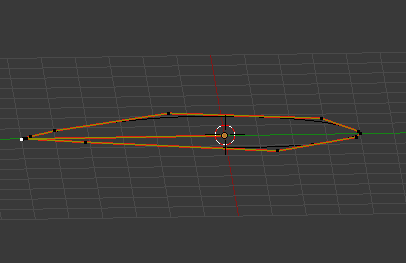# Spline curve from points: extra point at 0/0/0

Hi all,

I have a set of points and make a spline curve from them suing the following code.
I always have an extra point at 0/0/0. I guess its because the initialisation adds a default point which i can not figure out how to get rid of…

# create the Curve Datablock

``````curveData = bpy.data.curves.new(name, type='CURVE')
curveData.dimensions = '3D'
curveData.resolution_u = 2
``````
``````# map coords to spline
polyline = curveData.splines.new('NURBS')
for i, coord in enumerate(coords):
x,y,z = coord
polyline.points[i].co = (x, y, z, 1)
``````
``````if(tClosed):
polyline.use_cyclic_u = True

# create Object
curveOB = bpy.data.objects.new(name, curveData)
``````
``````# attach to scene and validate context
scn = bpy.context.scene